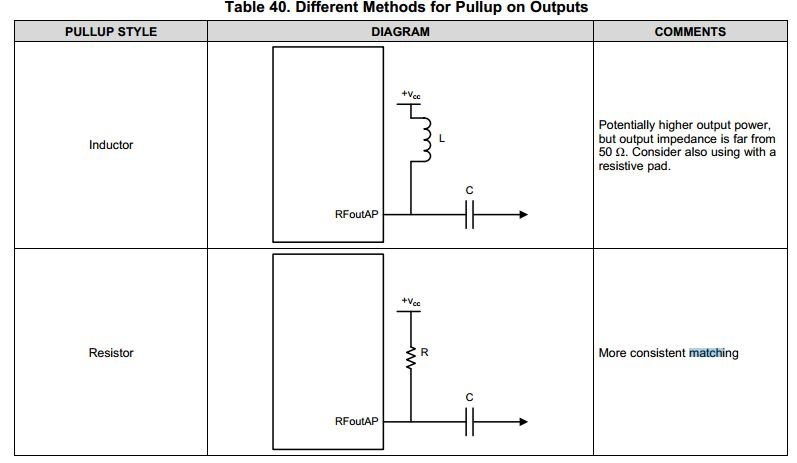• Resolved

# LMX2595: LMX2595 output matching

Part Number: LMX2595

There are two matching method  for the output matching of LMX295, one is using  pullup inductor to isloate the power and rf output,

the other is 50ohm resistor.

My quesiton is: if we choose the 50ohm resistor for pullingup on the outputs,  the real load of output is 25ohm,  which equals 50ohm(pullup resistor)//50ohm(RF load)

1. the real load for the output is 25ohm, there will be  mismatching betwwen the RFoutput and the load,  is 50ohm resistor pullup method reasonalbe?

2. due to the value of pullup resistor equals to 50ohm RF load, half of the energy will be consumered the pullup resistor,  this matching method is energy-wasting,

why do you recommend the resistor matching approaching in the design of  LMX2595?

Thank you!• Hi Jinfeng,

Right, the resistor pull-up approach will result in less output power. However, if your application requires a rather flat output power across the whole output range (10MHz to 20MHz), then the inductor pull-up approach is not the right choice.

In addition, resistor pull-up makes matching easier because the pull-up resistor makes the output impedance close to 50Ω

• In reply to Noel Fung:

Hi Noel,

How to understand the "the pull-up resistor makes the output impedance close to 50Ω"?

As I know if we choose the pull-up resistor method, the output impedance is approximately to 25ohm.

50ohm pull-up resistor parealles the 50ohm RF outputload equals to 25ohm, not the 50ohm.

• In reply to jinfeng tao:

Hi Jinfeng,

The output impedance of the buffer is very small, so the output impedance will very much look like the impedance of the pull-up resistor.

Datasheet has both the output power data using inductor or resistor pull-up, it should help you determine which pull-up is best suit to you.

• In reply to Noel Fung:

Hi Noel,

according to my understand,  the 50ohm pull  up resistor is shunt with output impedance of the buffer, That is to say,

50ohm // buffer output imedance == 50ohm // very small impedace ~=very small impedance of buffer,

So the output impedance is likely very small ,not the look like the impedance of the pull-up resistor.

Is my process of reasoning right?

• In reply to jinfeng tao:

Hi Jinfeng,

The output buffer is an open-drain buffer, the pull-up resistor is not a shunt to a standalone buffer amplifier.

Anyway, we recommend 50Ω pull-up or if you want higher output power, use inductor pull-up.

• In reply to Noel Fung:

Hi Noel,

OK, if i want higher output power, the inductor will be better choice, another quesiton:

if the value of inductor is vary the LO output frequency?

That is to say,  the value of inductor is different for 2.6GHz and 18GHz.

How to choose the value of inductor for the interset LO frequency?

Thank you!

• In reply to jinfeng tao:

Hi Jinfeng,

we have verified 1nH, 3.3nH and 10nH inductor, at 2GHz or above, we did not see big power difference. In other words, the inductor size does not matter.

We would recommend 1nH or 3.3nH inductor. We used:

LL1005-FH1N0S and LL1005-FH3N3S inductors.

• In reply to Noel Fung:

Hi  Noel,

Thank you very much for your detail explanation!